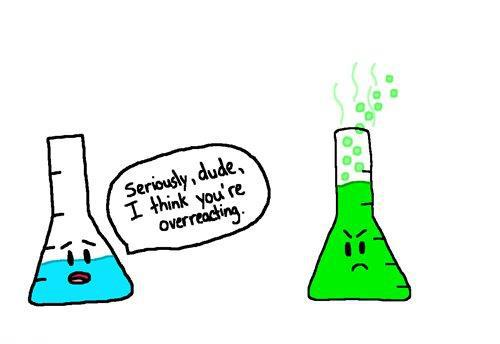### By the end of this unit students will be able to:

• Recognize the physical indicators of chemical reactions.
• Write chemical equations using the appropriate nomenclature and symbols.
• Satisfy the Law of Conservation of Mass while balancing chemical equations.
• Classify reactions (synthesis, decomposition, single-displacement, double-displacement, and combustion).
• Predict the products of a synthesis, decomposition, and combustion reactions.
• Use the activity series to predict the products of a single-displacement reaction.
• Use the solubility rules to determine the solubility of the products of a double-displacement reaction.
• Define endothermic and exothermic.
• Describe the factors that change the rate of the reaction.

Practice Balancing Equations at PhET

Explore Reaction Rates at PhET